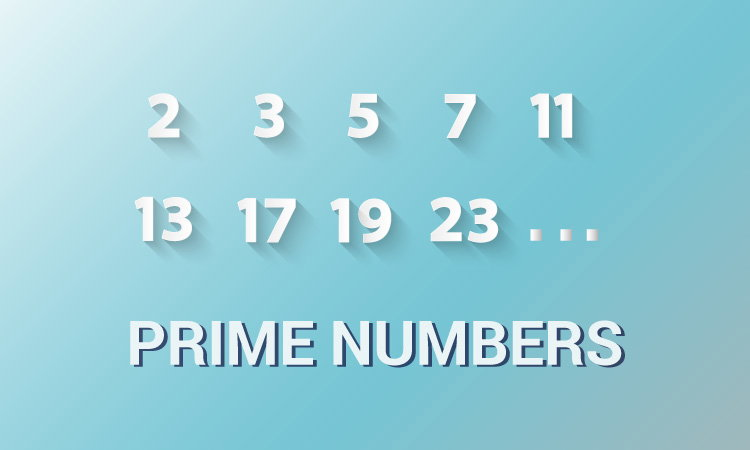# C Program to Display Prime Numbers Between Two Intervals

In this example, you will learn to print all prime numbers between two numbers entered by the user.To understand this example, you should have the knowledge of the following C programming topics:

## Display Prime Numbers Between two Intervals

``````#include <stdio.h>
int main() {
int low, high, i, flag;
printf("Enter two numbers(intervals): ");
scanf("%d %d", &low, &high);
printf("Prime numbers between %d and %d are: ", low, high);

while (low < high) {
flag = 0;

// if low is a non-prime number, flag will be 1
for (i = 2; i <= low / 2; ++i) {
if (low % i == 0) {
flag = 1;
break;
}
}

if (flag == 0)
printf("%d ", low);
++low;
}

return 0;
}
``````

Output

```Enter two numbers(intervals): 20
50
Prime numbers between 20 and 50 are: 23 29 31 37 41 43 47
```

In this program, the `while` loop is iterated ( `high-low-1`) times.

In each iteration, whether `low` is a prime number or not is checked, and the value of `low` is incremented by `1` until `low` is equal to `high`.

If the user enters the larger number first, the above program doesn't work as intended. You can solve this issue by swapping the numbers.

## Display Prime Numbers when Larger Number is Entered first

``````#include <stdio.h>
int main() {
int low, high, i, flag, temp;
printf("Enter two numbers(intervals): ");
scanf("%d %d", &low, &high);

// swap numbers if low is greather than high
if (low > high) {
temp = low;
low = high;
high = temp;
}

printf("Prime numbers between %d and %d are: ", low, high);
while (low < high) {
flag = 0;
for (i = 2; i <= low / 2; ++i) {
if (low % i == 0) {
flag = 1;
break;
}
}
if (flag == 0)
printf("%d ", low);
++low;
}

return 0;
}
``````

Visit this page to learn how you can display all the prime numbers between the two intervals by creating a user-defined function.

``````
``````